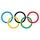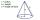# 22/7 circle

Calculate approximately area of a circle with radius 20 cm. When calculating π use 22/7.

Result

S =  1257.14 cm2

#### Solution:Leave us a comment of example and its solution (i.e. if it is still somewhat unclear...):Be the first to comment!## Next similar examples:

1. CableCable consists of 8 strands, each strand consists of 12 wires with diameter d = 0.5 mm. Calculate the cross-section of the cable.
2. AnnulusThe radius of the larger circle is 8cm, the radius of smaller is 5cm. Calculate the contents of the annulus.
3. Circle - easy 2The circle has a radius 6 cm. Calculate:
4. SidewalkThe city park is a circular bed of flowers with a diameter of 8 meters, around it the whole length is 1 meter wide sidewalk . What is the sidewalk area?
5. Diameter to areaFind the area of a circle whose diameter is 26cm.
6. Two circlesTwo circles with a radius 4 cm and 3 cm have a center distance 0.5cm. How many common points have these circles?
7. Bicycle wheelAfter driving 157 m bicycle wheel rotates 100 times. What is the radius of the wheel in cm?
8. CircleWhat is the radius of the circle whose perimeter is 6 cm?
9. Clock handsThe second hand has a length of 1.5 cm. How long does the endpoint of this hand travel in one day?
10. Circle - simpleThe circumference of a circle is 930 mm. How long in mm is its diameter?
11. CirclesFor the circle c1(S1; r1=146 cm) and c2(S2; r2 = 144 cm) is distance of centers |S1S2| = 295 cm. Determine the distance between the circles.
12. Area of ditchHow great content area will have a section of trapezoidal ditch with a width of 1.6 meters above and below 0.57 meters? The depth of the ditch is 2.08 meters.
13. The diagramThe diagram is a cone of radius 8cm and height 10cm. The diameter of the base is. ..
14. RoundingThe following numbers round to the thousandth:
15. Unknown number 11That number increased by three equals three times itself?
16. Simple equationSolve the following simple equation: 2. (4x + 3) = 2-5. (1-x)
17. Write 2Write 791 thousandths as fraction in expanded form.